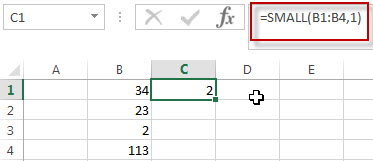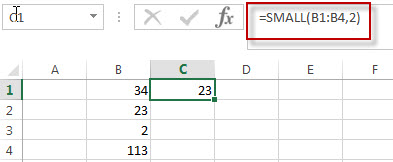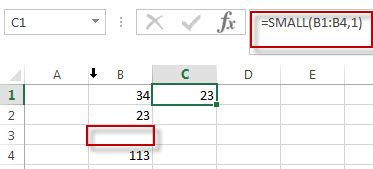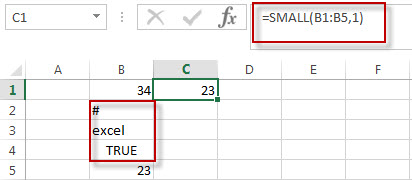# Excel SMALL Function

This post will guide you how to use Excel SMALL function with syntax and examples in Microsoft excel.

## Description

The Excel SMALL function returns the smallest numeric value from the numbers that you provided. Or returns the smallest value in the array. It also can be used to get the nth smallest value from a range of cells or an array. For example, the SMALL function can find the second smallest value, or third smallest value in a list or an array.

The SMALL function is a build-in function in Microsoft Excel and it is categorized as a Statistical Function.

The SMALL function is available in Excel 2016, excel 2013, excel 2010, excel 2007, excel 2003, excel XP, excel 2000, excel 2011 for Mac.

## Syntax

The syntax of the SMALL function is as below:

`= SMALL(array,nth)`

Where the SMALL function arguments are:

• array -This is a required argument.  An Array or a range of cells that contains numeric values from which you want to get the nth smallest value.
• nth – This is a required argument.  The position of the number that you want to return

Note:

• If the first argument is empty, then the small function returns #NUM! Error.
• If nth is less than or equal to 0 or if nth is greater than the number of values in array, the SMALL function returns the #NUM! Error.
• If nth is the number of values in array, then SMALL(array,1) returns the smallest value, and SMALL(array,n) returns the largest value.

## Excel SMALL Function Examples

The below examples will show you how to use Excel SMALL Function to get the smallest value form the numbers in a range.

#1 To get the smallest value from a range B1:B4, just using the following excel formula:

` =SMALL(B1:B4,1)`The above formula returns the smallest number from a range B1:B4, it returns 2.

2# To get the second smallest value from the Range B1:B4, you can use the following SMALL function:

`=SMALL(B1:B4,2)`The second argument nth in the above SMALL function is 2, so it will fetch the second smallest function in the range B1:B4. It returns 23.

3# To get the smallest value from the range B1:B4 that contains blank cells, enter into the following formula in the Cell C1:

`=SMALL(B1:B4,1)`The SMALL function will ignore blank cells in the Range. You will see that the Cell B3 is a blank cell, and it is ignored by the small function. The smallest value is still returned as 23 by the SMALL function.

4# To get the smallest value from the range B1:B5 that contains text string, specific character and logical values. Using the following SMALL formula:

`=SMALL(B1:B5,1)`The SMALL function will ignore values in the range B1:B5 that contains text string, specific character and logical value.

### More Excel Small Function Examples

• VLOOKUP Return Multiple Values Horizontally
You can create a complex array formula based on the INDEX function, the SMALL function, the IF function, the ROW function and the COLUMN function to vlookup a value and then return multiple corresponding values horizontally in Excel.…
• Copy and Paste Only Non-blank Cells
If you want only copy non-blank cells in a range in Excel, you need to select the non-blank cells firstly, then press Ctrl +C keys to copy the selected cells. So how to only select all non-blank cells in the selected range in your worksheet..…
• Find Missing Numbers in a Sequence in Excel
You can use an excel array formula based on the SMALL function, the IF function, the ISNA function, the MATCH function, and the ROW function to find missing numbers in a sequence…
• Find Closest Value or Nearest Value in a Range
You need to use an excel array formula based on the INDEX function, the MATCH function, the MIN function and the ABS function to find Closest Value or Nearest Value in a Range in Excel…